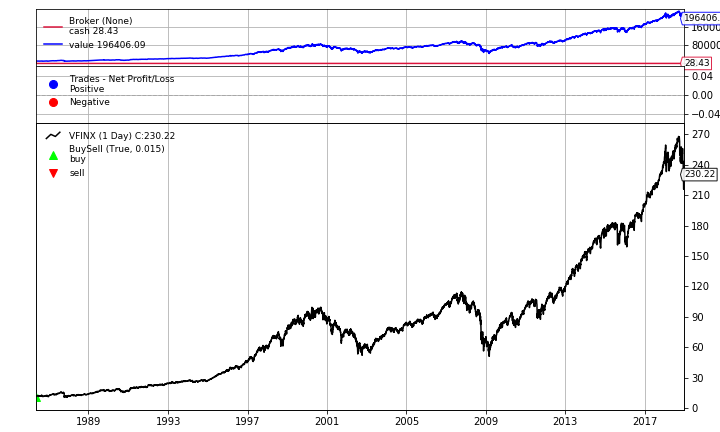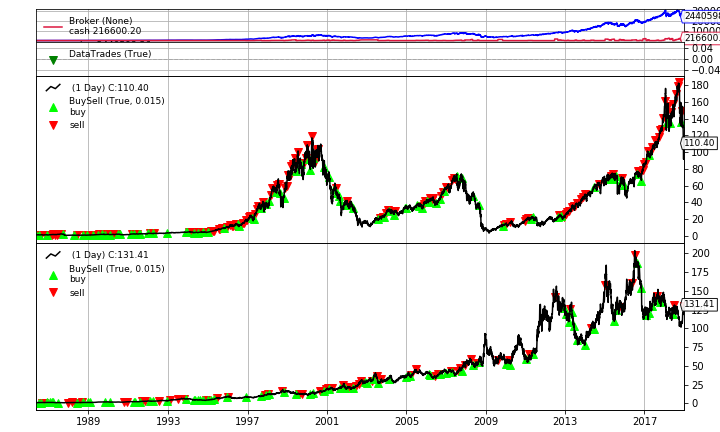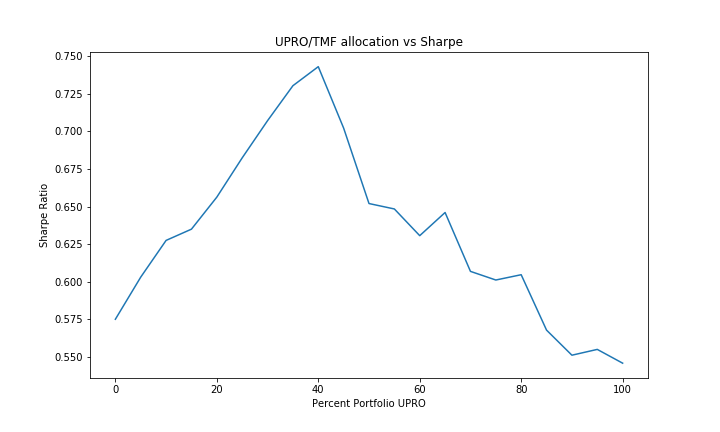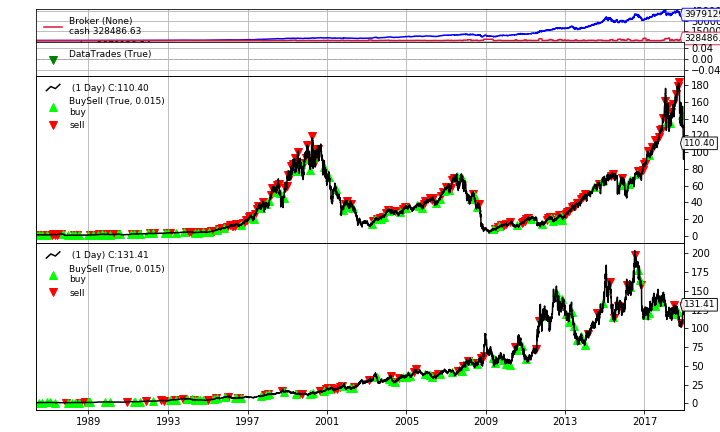In my last post we discussed simulation of the 3x leveraged S&P 500 ETF, UPRO, and demonstrated why a 100% long UPRO portfolio may not be the best idea. In this post we will analyze the simulated historical performance of another 3x leveraged ETF, TMF, and explore a leveraged variation of Jack Bogle’s 60 / 40 equity/bond allocation.

First lets import the libraries we need.

import pandas as pd
import datetime
import numpy as np
%matplotlib inline
import matplotlib.pyplot as plt
plt.rcParams["figure.figsize"] = (10, 6) # (w, h)


We’ll also need the sim_leverage function from my last post.

def sim_leverage(proxy, leverage=1, expense_ratio = 0.0, initial_value=1.0):
"""
Simulates a leverage ETF given its proxy, leverage, and expense ratio.

Daily percent change is calculated by taking the daily percent change of
the proxy, subtracting the daily expense ratio, then multiplying by the leverage.
"""
pct_change = proxy.pct_change(1)
pct_change = (pct_change - expense_ratio / 252) * leverage
sim = (1 + pct_change).cumprod() * initial_value
sim = initial_value
return sim


For this article we will be using two leveraged ETFs: UPRO, a 3x leveraged S&P 500 ETF featured in my last post, and TMF, a 3x leveraged U.S. Treasury 20+ Year Bond Index. We can create simulations for both UPRO and TMF using the following values:

ETF Expense Ratio Proxy Proxy Inception Date
UPRO 0.92% VFINX 08/31/1976
TMF 1.09% VUSTX 05/19/1986

We’ll use 05/19/1986 as our start date as that is when we’ll have data for both proxies.

start = datetime.datetime(1986, 5, 19)
end = datetime.datetime(2019, 1, 1)

upro_sim = sim_leverage(vfinx, leverage=3.0, expense_ratio=0.0092).to_frame("close")
tmf_sim = sim_leverage(vustx, leverage=3.0, expense_ratio=0.0109).to_frame("close")


## Backtesting

Before we look at a multi-asset strategy, lets see how each of the assets perform with a simple buy-and-hold strategy. For backtesting our strategies, we will be using Backtrader, a popular Python backtesting libray that also supports live trading.

In order for our data to work with Backtrader, we will have to fill in the open, high, low, and volume columns. For simplicity we will copy the close price to all columns, since we will only be trading at market close.

for column in ["open", "high", "low"]:
upro_sim[column] = upro_sim["close"]
tmf_sim[column] = tmf_sim["close"]

upro_sim["volume"] = 0
tmf_sim["volume"] = 0

upro_sim = bt.feeds.PandasData(dataname=upro_sim)
tmf_sim = bt.feeds.PandasData(dataname=tmf_sim)
vfinx = bt.feeds.YahooFinanceData(dataname="VFINX", fromdate=start, todate=end)


Now lets write our buy-and-hold strategy:

class BuyAndHold(bt.Strategy):
def next(self):
if not self.getposition(self.data).size:
self.order_target_percent(self.data, target=1.0)


We’ll also write a simple helper function that runs the backtest and returns important metrics. We will be using the Sharpe ratio to rate our strategies’ performance as it is a good way of measuring risk adjusted returns.

def backtest(datas, strategy, plot=False, **kwargs):
cerebro = bt.Cerebro()
for data in datas:
results = cerebro.run()
if plot:
cerebro.plot()
return (results.analyzers.drawdown.get_analysis()['max']['drawdown'],
results.analyzers.returns.get_analysis()['rnorm100'],
results.analyzers.sharperatio.get_analysis()['sharperatio'])


We’ll test our buy-and-hold strategy using VFINX, the S&P 500 ETF as our benchmark:

dd, cagr, sharpe = backtest([vfinx], BuyAndHold, plot=True)
print(f"Max Drawdown: {dd:.2f}%\nCAGR: {cagr:.2f}%\nSharpe: {sharpe:.3f}")Max Drawdown: 55.23%
CAGR: 9.55%
Sharpe: 0.652


We can see that, as intended, the strategy performs a single buy, then holds the asset for the remaining years.

Lets now run the buy-and-hold strategy on UPRO.

dd, cagr, sharpe = backtest([upro_sim], BuyAndHold)
print(f"Max Drawdown: {dd:.2f}%\nCAGR: {cagr:.2f}%\nSharpe: {sharpe:.3f}")

Max Drawdown: 97.11%
CAGR: 15.37%
Sharpe: 0.541


These numbers do not exactly match those of the last post because of the slightly shorter time period. We do, however, still have the massive 97% max drawdown.

Finally lets test buy-and-hold with TMF.

dd, cagr, sharpe = backtest([tmf_sim], BuyAndHold)
print(f"Max Drawdown: {dd:.2f}%\nCAGR: {cagr:.2f}%\nSharpe: {sharpe:.3f}")

Max Drawdown: 50.27%
CAGR: 16.12%
Sharpe: 0.575


In terms of Sharpe ratio, UPRO and TMF both underperform the S&P 500 ETF. Lets see what happens when we put them together!

### Multi Asset Allocation

Vanguard founder Jack Bogle had long advocated for an portfolio solely consisting of 60% U.S. Stocks and 40% bonds. We’ll use the same logic to create a 60 / 40 UPRO/TMF portfolio, rebalancing every 20 trading days. The exact allocation percent will remain a parameter so we can tinker with it later.

class AssetAllocation(bt.Strategy):
params = (
('equity',0.6),
)
def __init__(self):
self.UPRO = self.datas
self.TMF = self.datas
self.counter = 0

def next(self):
if  self.counter % 20 == 0:
self.order_target_percent(self.UPRO, target=self.params.equity)
self.order_target_percent(self.TMF, target=(1 - self.params.equity))
self.counter += 1


Now lets test it!

dd, cagr, sharpe = backtest([upro_sim, tmf_sim], AssetAllocation, plot=True, equity=0.6)
print(f"Max Drawdown: {dd:.2f}%\nCAGR: {cagr:.2f}%\nSharpe: {sharpe:.3f}")Max Drawdown: 61.62%
CAGR: 18.35%
Sharpe: 0.631


The Sharpe ratio is now higher than our S&P 500 benchmark. Lets see if we can improve it any more by optimizing the allocations.

### Optimization

Note: When optimizing parameters, one must be wary of overfitting. From the Backtrader website: “There is plenty of literature about Optimization and associated pros and cons. But the advice will always point in the same direction: do not overoptimize. If a trading idea is not sound, optimizing may end producing a positive result which is only valid for the backtested dataset.”

Lets run our backtest for all allocations of UPRO and TMF in 5% Intervals, and take note of each resulting Sharpe ratio. Backtrader does have built in parameter optimization functionality, it requires multithreading, which does not work within Jupyter Notebooks.

sharpes = {}
for perc_equity in range(0, 101, 5):
sharpes[perc_equity] = backtest([upro_sim, tmf_sim], AssetAllocation, equity=(perc_equity / 100.0))


This may take a minute or two. Once we have the results, we can graph how the portfolio allocation effects the Sharpe ratio, and find the optimal allocation.

series = pd.Series(sharpes)
ax = series.plot(title="UPRO/TMF allocation vs Sharpe")
ax.set_ylabel("Sharpe Ratio")
ax.set_xlabel("Percent Portfolio UPRO");
print(f"Max Sharpe of {series.max():.3f} at {series.idxmax()}% UPRO")Max Sharpe of 0.743 at 40% UPRO


In order to achieve the best Sharpe ratio in the backtest the best UPRO/TMF allocation is 40 / 60. Lets run one more backtest with this allocation.

dd, cagr, sharpe = backtest([upro_sim, tmf_sim], AssetAllocation, plot=True, equity=0.4)
print(f"Max Drawdown: {dd:.2f}%\nCAGR: {cagr:.2f}%\nSharpe: {sharpe:.3f}")Max Drawdown: 43.08%
CAGR: 20.13%
Sharpe: 0.743


## Conclusion

As we can see above, our simulated 40 / 60 UPRO/TMF portfolio more than doubled the annual returns of the S&P 500 ETF, all while producing a greater Sharpe ratio, and lesser max drawdown. While going long a single 3x leveraged ETF is probably not a good idea, a leveraged multi-asset strategy could provide significant gains while reducing risk.